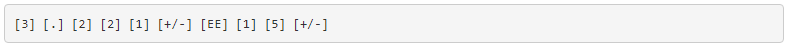# 4.5: Hand Calculator Use

## Scientific Notation with a Hand Calculator

To enter numbers in scientific notation into a hand calculator, there is usually a button marked “E” or “EE” used to enter the correct power of ten. For example, to enter the mass of a proton in grams (1.67 x 10-24grams) into a hand calculator, I would enter the following keystrokes:The [+/-] keystroke changes the sign of the power (24) into a -24. Some calculators allow the use of the subtraction key [-] to do this, but I prefer the “change sign” [+/-] key because its more consistent with the use of that key in other contexts.

If I wanted to enter a negative number in scientific notation into a hand calculator, I would have to be careful how I used the [+/-] key, lest I change the sign of the power and not the significant digit value. Pay attention to this example:

Number to be entered: -3.221 x 10-15:The first [+/-] keystroke changes the entry from 3.221 to -3.221; the second [+/-] keystroke changes the power from 15 to -15.

## Metric and Scientific Notation Display Modes

Displaying metric and scientific notation on a hand calculator is a different matter. It involves changing the display option from the normal “fixed” decimal point mode to the “scientific” or “engineering” mode. Your calculator manual will tell you how to set each display mode.

These display modes tell the calculator how to represent any number on the numerical readout. The actual value of the number is not affected in any way by the choice of display modes—only how the number appears to the calculator user. Likewise, the procedure for entering numbers into the calculator does not change with different display modes either. Powers of ten are usually represented by a pair of digits in the upper-right hand corner of the display, and are visible only in the “scientific” and “engineering” modes.

The difference between “scientific” and “engineering” display modes is the difference between scientific and metric notation. In “scientific” mode, the power-of-ten display is set so that the main number on the display is always a value between 1 and 10 (or -1 and -10 for negative numbers). In “engineering” mode, the powers-of-ten are set to display in multiples of 3, to represent the major metric prefixes. All the user has to do is memorize a few prefix/power combinations, and his or her calculator will be “speaking” metric!REVIEW:

• Use the [EE] key to enter powers of ten.
• Use “scientific” or “engineering” to display powers of ten, in scientific or metric notation, respectively.